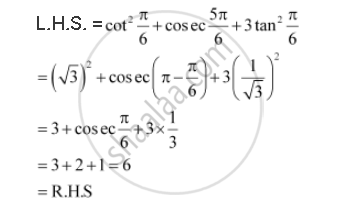CBSE (Arts) Class 11CBSE
Share

# Prove That Cossqrt2 Pi/6 + Cosec (5pi)/6 + 3 Tansqrt2 Pi/6 = 6 - CBSE (Arts) Class 11 - Mathematics

ConceptTrigonometric Functions of Sum and Difference of Two Angles

#### Question

Prove that  cos^2  pi/6 + cosec (5pi)/6 + 3 tan^2  pi/6 = 6

#### SolutionIs there an error in this question or solution?

#### APPEARS IN

NCERT Solution for Mathematics Textbook for Class 11 (2018 to Current)
Chapter 3: Trigonometric Functions
Q: 3 | Page no. 73

#### Video TutorialsVIEW ALL 

Solution Prove That Cossqrt2 Pi/6 + Cosec (5pi)/6 + 3 Tansqrt2 Pi/6 = 6 Concept: Trigonometric Functions of Sum and Difference of Two Angles.
S